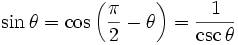# Sine

Sine is a trigonometric function describing the ratio between the opposite side and the hypotenuse in a right triangle. As a function it is usually abbreviated sin.

The sine of something is generally calculated in radians, a means of measuring angles determined by the measure of the arc of a circle cut by such an angle, divided by the length of the radius of the circle, so that a full 360° angle would be 2π radians, 180° would be π, 90° would be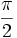radians, and so on.

## Sine Graph

The basic sine graph,, where x is in radians, is as follows:

x values y values
0 0
π/2 1
π 0
3π/2 -1
0That would be a full period of the graph - as 2π radians is equivalent to one circle. The graph continues in that pattern.

## Famous Sines

Because of the known relationship of the sides of a right triangle with the angles 30°, 45°, or 60°, certain values of sine are calculable using exact values in the form of square roots and fractions involving square roots. These include: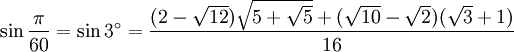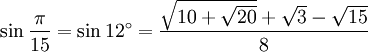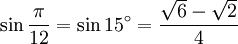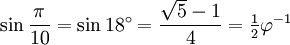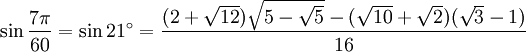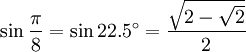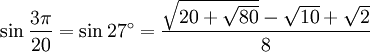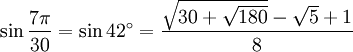## Relationship to cosine and cosecant

A mathematical identity involving sine is: